Solar Energy Local
Solar Energy & Solar Power Resources

# Solar Energy in Newton, MA

## Solar Energy in Newton

The month of July has the highest historical solar radition values in Newton with an average of 5.99 kWh/m2/day. [More]

## Solar Panels in Newton

Solar panels in Newton that are tilted towards the equator at an angle equal to the latitude will produce the maximum solar energy output. [More]

## Sunrise & Sunset in Newton

The total length of the day in Newton, MA today (March 27, 2023) will be 12 hours and 29 minutes. [More]## Solar Power in Newton, MA

### Solar Energy Data in Newton, MA

See the chart below to see how different types of solar panel installations compare in Newton.

* Amounts listed in kWh/m2/day (kilowatt hours per square meter per day).
* See glossary & definitions below.

### Solar Energy Analysis for Newton, MA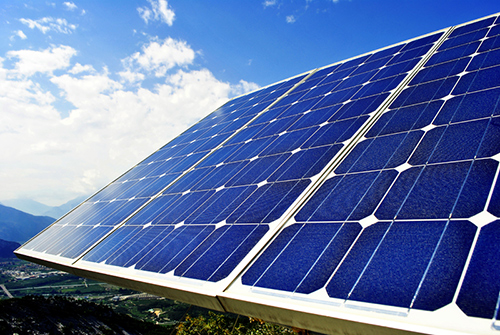Based on historical Newton, MA data, solar panels that are tilted towards the equator at an angle equal to the latitude will produce the maximum solar energy output in Newton. 

Newton has an average monthly Global Horizontal Irradiance (GHI) of 3.83 kilowatt hours per square meter per day (kWh/m2/day), which is approximately equal to the average monthly Direct Normal Irradiance (DNI) of 3.83 kWh/m2/day. 

Solar installations in Newton that are always titled at the latitude of Newton (Average Tilt at Latitude or ATaL) average 4.57 kWh/m2/day, or about 19% greater than the average monthly GHI of 3.83 kWh/m2/day and approximately 19% greater than the average monthly DNI of 3.83 kWh/m2/day. 

## Solar Energy Glossary

Global Horizontal Irradiance: The total amount of solar radiation that is received per unit area by a surface that is always positioned in a horizontal manner.

Direct Normal Irradiance: The total amount of solar radiation received per unit area by a surface that is always perpendicular to the sun rays that come in a straight line from the direction of the sun at its current position in the sky.

### Average Tilt at Latitude (ATaL)

Average Tilt at Latitude: The total amount of solar radiation received per unit area by a surface that is tilted toward the equator at an angle equal to the current latitude. ATaL will often produce the optimum energy output.

## Solar Radiation Levels in Newton

### Solar Radiation Data in Newton, MA

See the chart below for monthly solar radiation levels in Newton.

* Amounts listed in kWh/m2/day (kilowatt hours per square meter per day)

### Solar Radiation Analysis for Newton, MA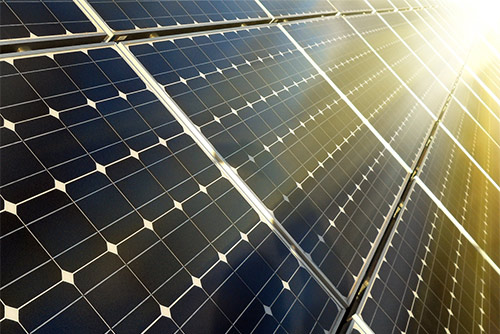Newton, MA has a average annual solar radiation value of 4.92 kilowatt hours per square meter per day (kWh/m2/day). 

The month with the highest historical solar radition values in Newton is July with an average of 5.99 kWh/m2/day, followed by August at 5.78 kWh/m2/day and June at 5.65 kWh/m2/day. 

The three months that historically average the lowest average solar radiation levels in Newton (Massachusetts) are December with an average of 3.25 kWh/m2/day, followed by November with an average of 3.82 kWh/m2/day and January at 4 kWh/m2/day. 

## Solar Power Comparison: Newton vs. the U.S.

### Solar Power Levels in Newton

The average monthly solar radiation level in Newton, MA, of 4.92 kilowatt hours per square meter per day (kWh/m2/day) is approximately 25% greater than the average level of 3.93 kWh/m2/day in a city with historically low levels (WA) and is approximately 26% less than the average level of 6.61 kWh/m2/day in a city with historically high levels (NV). 

→ Values listed as 0 (zero) are not available (N/A).

### Solar Power: Newton vs. the U.S.

Below is a month-by-month comparison of how average Newton solar radiation levels compare to average levels in a city with historcially high levels (NV) and a city with historically low levels (WA). 

→ k/m/d = kWh/m2/day = kilowatt hours per square meter per day.
→ Values listed as 0 (zero) are not available (N/A).

## Solar Data & Statistics for Newton

### Sunrise / Sunset in Newton

• Sunrise/Sunset for March 27, 2023 
• Sunrise: 5:35 am
• Sunset: 6:04 pm
• Length of Day: 12:29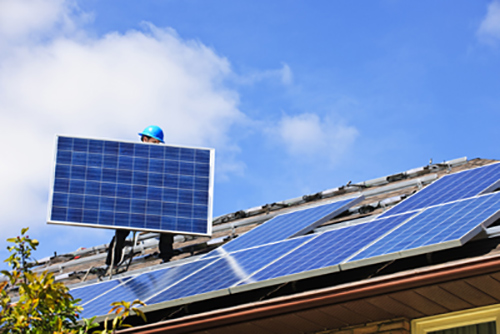### Best Months for Solar in Newton

• Top Solar Power Months Historically 
• Solar Radiation July (5.99 kWh/m2/day)
• ATaL: July (5.46 kWh/m2/day)
• DNI: July (4.53 kWh/m2/day)
• GHI: July (5.84 kWh/m2/day)

### Solar Panel Installs in Newton

We do not currently have data on solar panel installations in the city of Newton, MA at this current time.

## Solar Power Output in Newton

### The Power of Solar in Newton, MA

Monthly AC solar system output averages for Newton (ma). Month-by-month solar power, as ranked by AC output, in Newton. 

* Amounts listed in kWhac (kilowatt hours (AC))

### Solar Output Analysis for Newton, MA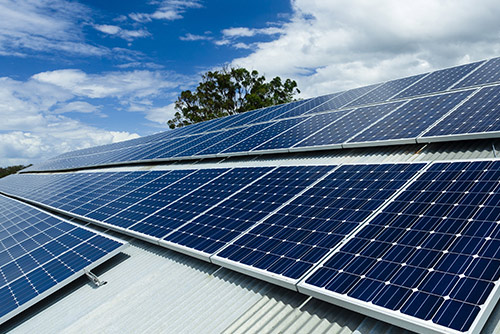Newton (MA) has a average annual solar AC output value of 5471.67 kilowatt hours (AC). 

The month with the highest historical solar power output in Newton is July with an average of 541.29 kWhac, followed by August at 522.35 kWhac and May at 509.3 kWhac. 

The three months that historically average the lowest average solar output levels in Newton (Massachusetts) are December with an average of 324.93 kWhac, followed by November with an average of 361.61 kWhac and January at 406.24 kWhac. 

## Cost of Utilities in Newton

### Newton Electricity Costs

Sorry, Newton residential electricity prices are currently unavailable.

### Solar Power Poll: Newton

Vote now: Given all of the data and analysis provided for Newton, is solar power a good choice for residents of this city?

Yes, solar power is a great option
No, solar power isn't a good option

### Newton Natural Gas Costs

Sorry, Massachusetts residential natural gas prices are currently unavailable.

## Weather Forecast for Newton, MA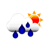Mon Mar 27 55°F Moderate Rain Min: 36°F Max: 57°F Gentle Breeze: 8 mps ESE Precip.: Chance of Rain Humidity: 35% Cloud cover: 4%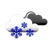Tue Mar 28 38°F Rain And Snow Min: 36°F Max: 41°F Gentle Breeze: 8 mps ENE Precip.: Chance of Rain Humidity: 97% Cloud cover: 100%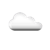Wed Mar 29 44°F Scattered Clouds Min: 31°F Max: 50°F Gentle Breeze: 10 mps SSW Precip.: n/a Humidity: 40% Cloud cover: 33%Thu Mar 30 35°F Light Snow Min: 29°F Max: 39°F Moderate breeze: 15 mps WNW Precip.: Chance of Snow Humidity: 28% Cloud cover: 1%Fri Mar 31 43°F Light Rain Min: 29°F Max: 50°F Gentle Breeze: 9 mps S Precip.: Chance of Rain Humidity: 32% Cloud cover: 62%Sat Apr 1 47°F Moderate Rain Min: 40°F Max: 56°F Moderate breeze: 14 mps SW Precip.: Chance of Rain Humidity: 98% Cloud cover: 100%

The city of Newton, MA in Middlesex County has an approximate population of 85,146. 

### Newton, MA Solar Energy

To learn more about solar energy and solar power Newton, MA, or for more solar-related resources for Newton, visit the U.S. Energy Information Administration.

### Did you know?

Various missions to space by different countries are known to utilize solar energy as a main power source.

Go

## References

[ X ]

#### Is solar right for your home?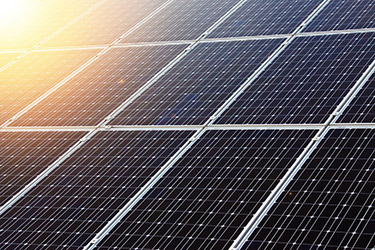Take the first step toward powering your home with solar energy.

Start now with an online solar savings estimate.